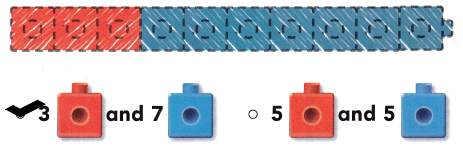# Texas Go Math Kindergarten Lesson 5.4 Answer Key Ways to Make 10

Refer to our Texas Go Math Kindergarten Answer Key Pdf to score good marks in the exams. Test yourself by practicing the problems from Texas Go Math Kindergarten Lesson 5.4 Answer Key Ways to Make 10.

## Texas Go Math Kindergarten Lesson 5.4 Answer Key Ways to Make 10

Unlock the Problem

DIRECTIONS: Use cubes of two colors to show different ways to make 10. Color to show the ways.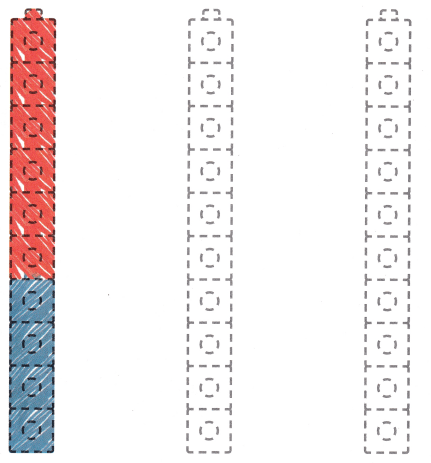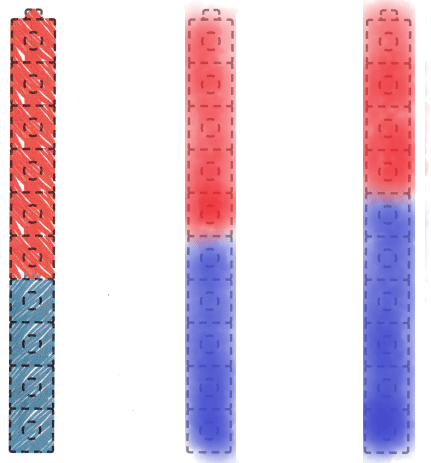Explanation:
I used cubes of two colors to show different ways to make 10. I colored 5 cubes red and 5 blue to show one way and 4 cubes red and 6 blue to show another way to make 10.

Try Another Problem

DIRECTIONS: 1. Count and tell how many cubes of each color there are. Trace the numbers. 2-3. Color cubes blue to match the number. Color the other cubes red to make 10. Write how many cubes Write how many cubes in all.

Question 1.Explanation:
I counted the number of cubes.There are 9 blue cubes, 1 red cubes and 10 cubes in all.

Question 2.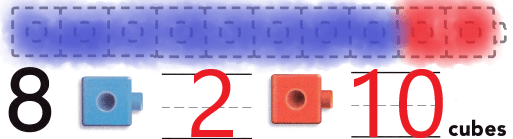Explanation:
I colored 8 cubes blue to match the number 8 and colored the other 2 cubes red to make 10.
There are 10 cubes in all.

Question 3.Explanation:
I colored 7 cubes blue to match the number 7 and colored the other 3 cubes red to make 10.
There are 10 cubes in all.

Share and Show

DIRECTIONS: 4-6. Color cubes blue to match the number. Color the other cubes red to make 10. Write how many red cubes. Write how many cubes in all.

Question 4.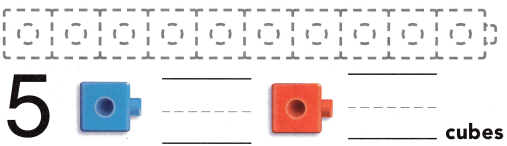Explanation:
I colored 5 cubes blue to match the number 5 and colored the other 5 cubes red to make 10.
There are 10 cubes in all.

Question 5.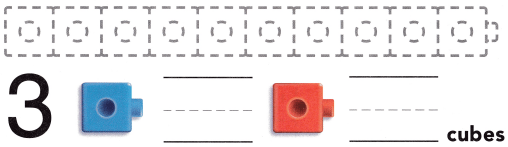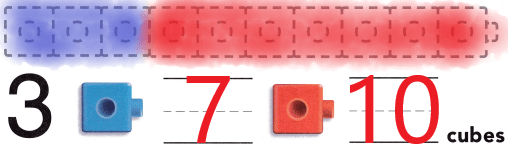Explanation:
I colored 3 cubes blue to match the number 3 and colored the other 7 cubes red to make 10.
There are 10 cubes in all.

Question 6.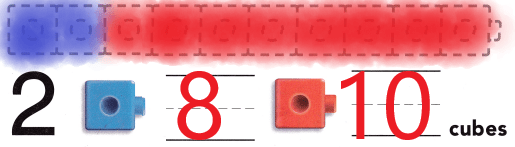Explanation:
I colored 2 cubes blue to match the number 2 and colored the other 8 cubes red to make 10.
There are 10 cubes in all.

HOME ACTIVITY • Ask your child to show a set of 10 objects. Have him or her write numbers to show the set.

DIRECTIONS: Choose the correct answer. 7. Ella has ten toy trucks. Which number shows how many trucks she has? 8. How many more cubes should you color to make 10? 9. How is 10 shown in the cube train?

Question 7.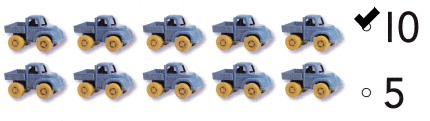Explanation:
I marked the number 10 as there are 10 toy truks.

Question 8.Explanation:
I marked 3 as we need to color 3 more cubes to make 10.

Question 9.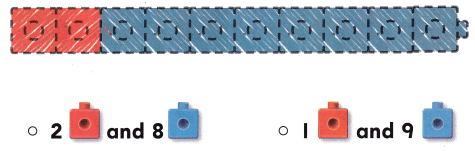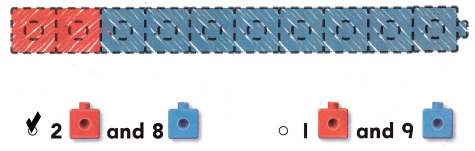Explanati:
I marked the option that tells that there are 2 red cubes and 8 blue cubes in the above cube train.

### Texas Go Math Kindergarten Lesson 5.4 Homework and Practice Answer Key

DIRECTIONS: 1-3. Use blue to color the cubes to match the number. Use red to color the other cubes. Write how many red cubes. Write how many cubes in all.

Question 1.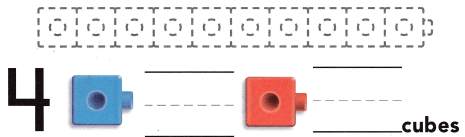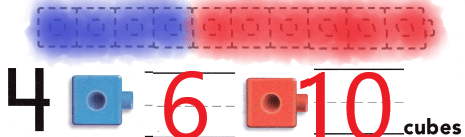Explanation:
I colored 4 cubes blue to match the number 4 and colored the other 6 cubes red to make 10.
There are 10 cubes in all.

Question 2.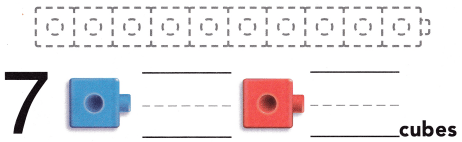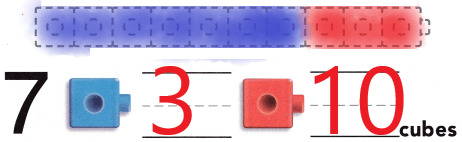Explanation:
I colored 7 cubes blue to match the number 7 and colored the other 3 cubes red to make 10.
There are 10 cubes in all.

Question 3.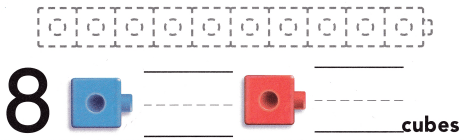Explanation:
I colored 8 cubes blue to match the number 8 and colored the other 2 cubes red to make 10.
There are 10 cubes in all.

DIRECTIONS: Choose the correct answer. 4-5. How many more cubes should you color to make 10? 6. How is 10 shown on the cube train?

Lesson Check

Question 4.Explanation:
I marked the number 5 as we need to color 5 more cubes to make 10.

Question 5.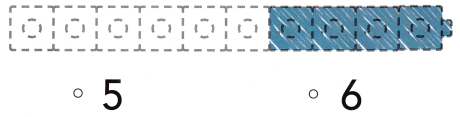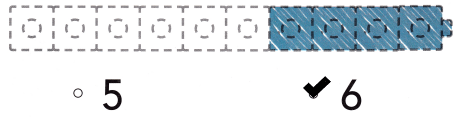Explanation:
I marked the number 6 as we need to color 6 more cubes to make 10.

Question 6.